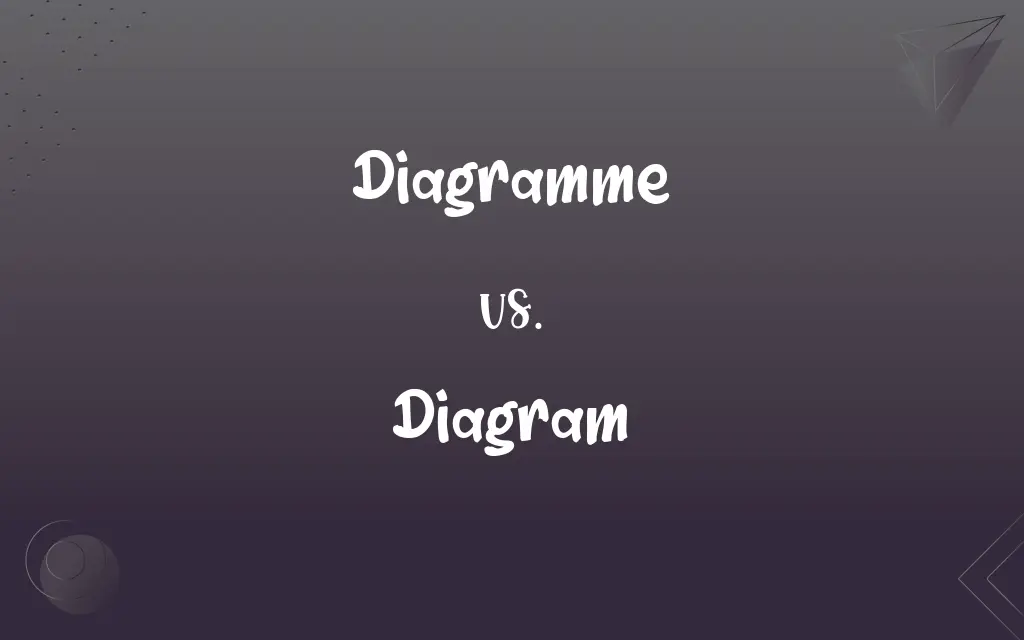# Diagramme vs. Diagram## Diagramme and Diagram Definitions

#### Diagramme

Archaic form of diagram

#### Diagram

A plan, sketch, drawing, or outline designed to demonstrate or explain how something works or to clarify the relationship between the parts of a whole.

#### Diagram

(Mathematics) A graphic representation of an algebraic or geometric relationship.

#### Diagram

A chart or graph.

#### Diagram

To indicate or represent by or as if by a diagram.

#### Diagram

A plan, drawing, sketch or outline to show how something works, or show the relationships between the parts of a whole.
Electrical diagrams show device interconnections.

#### Diagram

A graph or chart.

#### Diagram

(category theory) A functor from an index category to another category. The objects and morphisms of the index category need not have any internal substance, but rather merely outline the connective structure of at least some part of the diagram's codomain. If the index category is J and the codomain is C, then the diagram is said to be "of type J in C".

#### Diagram

(transitive) To represent or indicate something using a diagram.

#### Diagram

(UK) To schedule the operations of a locomotive or train according to a diagram.

#### Diagram

A figure or drawing made to illustrate a statement, or facilitate a demonstration; a plan.

#### Diagram

Any simple drawing made for mathematical or scientific purposes, or to assist a verbal explanation which refers to it; a mechanical drawing, as distinguished from an artistical one.